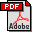TRACEMIN-Fiedler: A Parallel Algorithm for Computing the Fiedler Vector
Murat Manguoglu (Purdue University)
Eric Cox (Purdue University)
Faisal Saied (Purdue University)
Ahmed Sameh (Purdue University)
Abstract:
The eigenvector corresponding to the second smallest eigenvalue of the Laplacian of a graph, known as the Fiedler vector, has a number of applications in areas that include matrix reordering, graph partitioning, protein analysis, data mining, machine learning, and web search. The computation of the Fiedler vector has been regarded as an expensive process as it involves solving a large eigenvalue problem. We present a novel and efficient parallel algorithm for computing the Fiedler vector of large graphs based on the Trace Minimization algorithm. We compare the parallel performance of our method with a multilevel scheme, designed specifically for computing the Fiedler vector, which is implemented in routine MC73 FIEDLER of the Harwell Subroutine Library (HSL).
Keywords:
Parallel and Distributed Computing, Numerical Algorithms for CS&EDownload the fullpaper